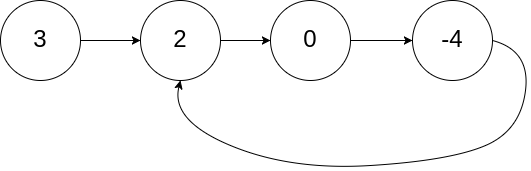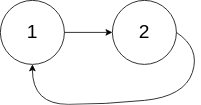## Algorithm

Problem Name: 142. Linked List Cycle II

Given the `head` of a linked list, return the node where the cycle begins. If there is no cycle, return `null`.

There is a cycle in a linked list if there is some node in the list that can be reached again by continuously following the `next` pointer. Internally, `pos` is used to denote the index of the node that tail's `next` pointer is connected to (0-indexed). It is `-1` if there is no cycle. Note that `pos` is not passed as a parameter.

Do not modify the linked list.

Example 1:```Input: head = [3,2,0,-4], pos = 1
Output: tail connects to node index 1
Explanation: There is a cycle in the linked list, where tail connects to the second node.
```

Example 2:```Input: head = [1,2], pos = 0
Output: tail connects to node index 0
Explanation: There is a cycle in the linked list, where tail connects to the first node.
```

Example 3:```Input: head = , pos = -1
Output: no cycle
Explanation: There is no cycle in the linked list.
```

Constraints:

• The number of the nodes in the list is in the range `[0, 104]`.
• `-105 <= Node.val <= 105`
• `pos` is `-1` or a valid index in the linked-list.

## Code Examples

### #1 Code Example with C Programming

```Code - C Programming```

``````
struct ListNode *detectCycle(struct ListNode *head) {
struct ListNode *n, *nn;

do {
n = n->next;           // one step
nn = nn->next;
if (nn) nn = nn->next;  // two steps
} while (nn && n != nn);

if (!nn) return NULL;

// there is a cycle
while (n != nn) {
n = n->next;
nn = nn->next;
}

return n;
}``````
Copy The Code &

Input

cmd
head = [3,2,0,-4], pos = 1

Output

cmd
tail connects to node index 1

### #2 Code Example with C++ Programming

```Code - C++ Programming```

``````
class Solution {
public:
while(two && two->next){
one = one->next;
two = two->next->next;
if(one == two){
meet = one;
break;
}
}
if(!two || !two->next) return NULL;
while(p != meet){
p = p->next;
meet = meet->next;
}
return p;
}
};
``````
Copy The Code &

Input

cmd
head = [3,2,0,-4], pos = 1

Output

cmd
tail connects to node index 1

### #3 Code Example with Java Programming

```Code - Java Programming```

``````
public class Solution {
while (fast != null && fast.next != null) {
slow = slow.next;
fast = fast.next.next;
if (slow == fast) {
break;
}
}
if (fast == null || fast.next == null) {
return null;
}
while (slow != fast) {
slow = slow.next;
fast = fast.next;
}
return slow;
}
}
``````
Copy The Code &

Input

cmd
head = [1,2], pos = 0

Output

cmd
tail connects to node index 0

### #4 Code Example with Javascript Programming

```Code - Javascript Programming```

``````
while(fast !== null && fast.next !== null) {
fast = fast.next.next
slow = slow.next
if(fast === slow) {
while(slow !== start) {
slow = slow.next
start = start.next
}
return start
}
}
return null
};
``````
Copy The Code &

Input

cmd
head = [1,2], pos = 0

Output

cmd
tail connects to node index 0

### #5 Code Example with Python Programming

```Code - Python Programming```

``````
class Solution:
def detectCycle(self, head: ListNode) -> ListNode:
fast = slow = root = head
while fast and fast.next:
slow = slow.next
fast = fast.next.next
if slow == fast:
while root != slow:
root = root.next
slow = slow.next
return root
``````
Copy The Code &

Input

cmd
head = , pos = -1

Output

cmd
no cycle